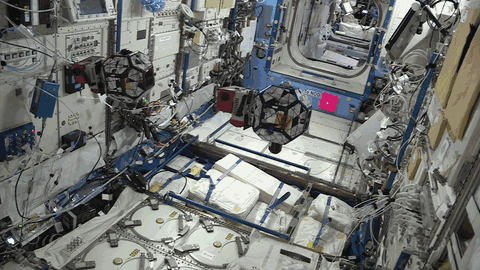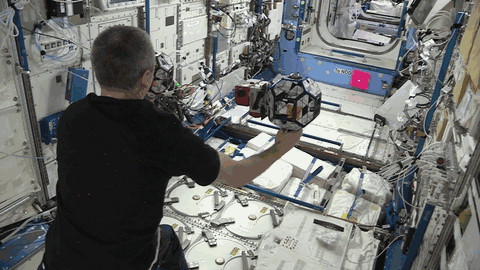# TS106 T2: Close Approach (GM)

Overview: One of the two planned close proximity scenarios for comparing the filtering- and the smoothing-based estimation approaches. The secondary satellite starts without any visual lock on the primary satellite, in order to test the cooperative localization portion of the proposed approach. The primary satellite performs a couple of close inspection approaches upon the secondary sat, finishing by having the secondary slew and obtain a target lack on the primary, trying to close the estimation loop between the two agents.

• test executed a total of 2 times (global runs 2, 14)

Information in debug vectors:

• float (8 vals):
• 0 = `ownGtsamState.pos` : x-position
• 1 = `ownGtsamState.pos` : y-position
• 2 = `ownGtsamState.pos` : z-position
• 3 = `ownGtsamState.quat` : x-component
• 4 = `ownGtsamState.quat` : y-component
• 5 = `ownGtsamState.quat` : z-component
• 6 = `ownGtsamState.quat` : w-component
• 7 = `test_time/100.0f` : test time
• short signed (16 vals):
• 0 = `test_time/100.0f` : test time
• 1 = `ctrlStateTarget[POS_X]*1000` : scaled x-position
• 2 = `ctrlStateTarget[POS_Y]*1000` : scaled y-position
• 3 = `ctrlStateTarget[POS_Z]*1000` : scaled z-position
• 4 = `ctrlStateTarget[VEL_X]*1000` : scaled x-velocity
• 5 = `ctrlStateTarget[VEL_Y]*1000` : scaled y-velocity
• 6 = `ctrlStateTarget[VEL_Z]*1000` : scaled z-velocity
• 7 = `ctrlStateTarget[QUAT_1]*1000` : scaled x-component
• 8 = `ctrlStateTarget[QUAT_2]*1000` : scaled y-component
• 9 = `ctrlStateTarget[QUAT_3]*1000` : scaled z-component
• 10 = `ctrlStateTarget[QUAT_4]*1000` : scaled w-component
• 11 = `ctrlStateTarget[RATE_X]*1000` : scaled x-rate
• 12 = `ctrlStateTarget[RATE_Y]*1000` : scaled x-rate
• 13 = `ctrlStateTarget[RATE_Z]*1000` : scaled x-rate
• 14 = `-1` : unused
• 15 = `-1` : unused
• short unsigned (16 vals):
• thruster firing times

## Run 1, Global 2

OVHD ISS Feed### Plots

Steps to follow to produce the desired plots:

• MATLAB

## Run 2, Global 14

OVHD ISS Feed